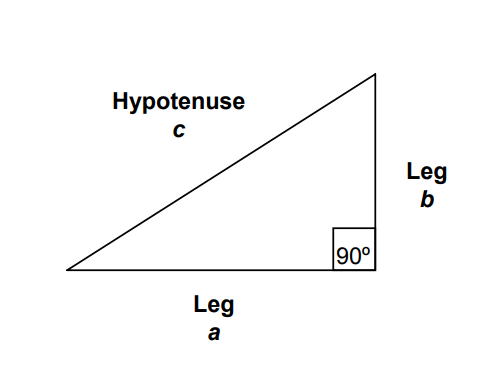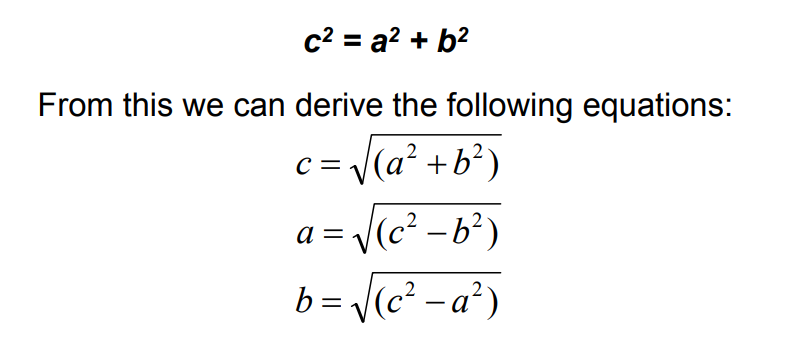# Calculate Hypotenuse of a Right Triangle

The hypotenuse calculator is an online tool to calculate hypotenuse of a triangle. Pythagoras’ theorem relates the lengths of the sides of a right-angled triangle. In any right-angled triangle, the side opposite the right-angle is called the hypotenuse. If the length of the hypotenuse of a right-angled triangle is c and the lengths of the other sides are a and b; the Pythagoras’ theorem states that the square of the hypotenuse, (c2), is equal to the sum of the squares of the other two sides, (a2 + b2).## How to find Hypotenuse

To find the hypotenuse, add the squares of the other sides, then take the square root.

To find a shorter side, subtract the squares of the other sides, then take the square root.

### What is the hypotenuse of a triangle?

A hypotenuse is the longest side of a right triangle. It's the side that is opposite to the right angle (90°). Since the hypotenuse is always opposite the right angle, it is very easy to identify. The other two sides are referred to as the legs of the triangle. Two notations are typically given to the legs: the opposite side, and the adjacent side.

The length of any side of a right triangle can be computed if the lengths of the other two sides are known. This property is derived from the Pythagorean Theorem.

#### Pythagorean TheoremIn a right triangle, the square of the length of the hypotenuse is equal to the sum of the squares of the lengths of the legs. Conversely, if the square of the length of the hypotenuse is equal to the sum of the squares of the lengths of the legs of a triangle, then the triangle is a right triangle, where the 90º angle is opposite the longest side.